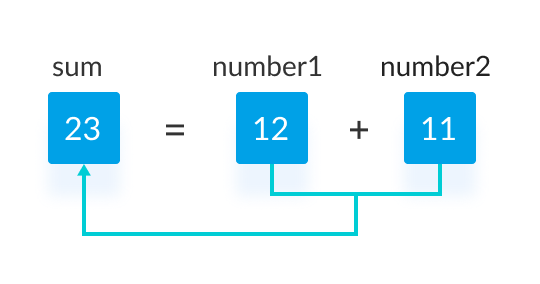# C Program to Add Two Integers

In this example, the user is asked to enter two integers. Then, the sum of these two integers is calculated and displayed on the screen.

To understand this example, you should have the knowledge of the following C programming topics:

## Program to Add Two Integers

``````#include <stdio.h>
int main() {

int number1, number2, sum;

printf("Enter two integers: ");
scanf("%d %d", &number1, &number2);

// calculating sum
sum = number1 + number2;

printf("%d + %d = %d", number1, number2, sum);
return 0;
}
``````

Output

```Enter two integers: 12
11
12 + 11 = 23
```

In this program, the user is asked to enter two integers. These two integers are stored in variables number1 and number2 respectively.

``````printf("Enter two integers: ");
scanf("%d %d", &number1, &number2);
``````

Then, these two numbers are added using the `+` operator, and the result is stored in the sum variable.

``````sum = number1 + number2;
``````Finally, the `printf()` function is used to display the sum of numbers.

``````printf("%d + %d = %d", number1, number2, sum);
``````